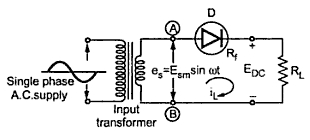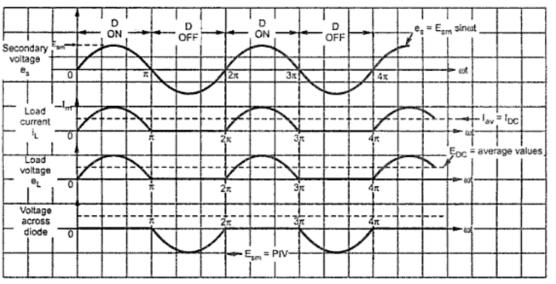### Half Wave Rectifier : Part1

In half wave rectifier , rectifying element conducts only during positive half cycle of input a.c. supply. The negative half cycles of a.c. supply are eliminated from the output.
This rectifier circuit consists of resistive load, rectifying element, i.e. p-n junction diode, and the source of a.c. voltage, all connected in series. The circuit diagram is shown in the Fig. 1. Usually, the rectifier circuits are operated from a.c. mains supply. To obtain the desired d.c. voltage across the load, the a.c. voltage is applied to rectifier circuit using suitable step-up or step-down transformer, mostly a step-down one, with necessary turns ratio.Fig. 1  Half wave rectifier
The input voltage to the half-wave rectifier circuit shown in the Fig. 1 is a sinusoidal a.c. voltage, having a frequency which is the supply frequency, 50 Hz.
The transformer decides the peak value of the secondary voltage. If  N1 are the primary number of turns and N2 are the secondary number of turns and Epm is the peak value of the primary voltage then,
where         Esm   = The peak value of the secondary a.c. voltage.
As the return of Esm is sinusoidal the instantaneous value will be,
es = Esm  sinωt
ω = 2πf
f = Supply frequency
Let Rf represents the forward resistance of the diode. Assume that, under reverse biased condition, the diode acts almost as an open circuit, conducting no current.
1.1 Operation of the Circuit
During the positive half cycle of secondary a.c. voltage, terminal (A) becomes positive with resoect to terminal (B). The diode is forward biased and the current flows in the circuit in the clockwise direction, as shown in the Fig. 2(a). The current will flow for almost full positive half cycle. This current is also flowing through load resistance RL hence denoted as IL, the load current.
During negative half cycle when terminal (A) is negative with respect to terminal (B), diode becomes reverse biased. Hence no current flows in the circuit as shown in the Fig. 2(b). Thus the circuit current, which is also the load current, is in the form of half sinusoidal pulses.Fig. 2

The load voltage, being the product of load current and load resistance, will also be in the form of half sinusoidal pulses. The different waveforms are illustrated in Fig. 3.Fig. 3  Load current and load voltage waveforms for half wave rectifier

The d.c. output waveform is expected to be a straight line but the half wave rectifier gives output in the form of positive sinusoidal pulses. Hence the output is called pulsating d.c. It is discontinuous in nature. Hence it is necessary to calculate the average value of load current and average value of output voltage.
1.2 Average DC Load Current ()
The average or dc value of alternating current is obtained by integration.
For finding out the average value of an alternating waveform, we have to determine the area under the curve over one complete cycle i.e. from 0 to 2π and then dividing it by the base i.e. 2π.
Mathematically, current waveform can be described as,
IL = Im sin t                  for 0 ≤ ωt ≤ π
IL = 0                        for π ≤ ωt ≤ 2π
Where  Im = Peak value of load current
As no current flows during negative half cycle of a.c. input voltage, i.e. between ωt = π to ωt = 2π, we change the limits of integration.
Applying Kirchhoff's voltage law we can write,
Where Rs = Resistance of secondary winding of transformer. If Rs is not given it should be neglected while calculating Im.
1.3 Average DC Load Voltage (EDC)
It is the product of average D.C. load current and the load resistance RL.
The winding resistance Rs and forward diode resistance Rf are practically very small compared to RL.
But as Rf and Rs are small compared to RL, (Rf +Rs )/RL is negligibly small compared to 1. So neglecting it we get,
Note : When Rf and Rs are finite, calculate Im, then IDC and from that calculate as .
Note : Do not calculate EDC as Esm/π directly for finite Rf and Rs. Calculate Im1 then and then EDC = IDC RL .
1.4 R.M.S. Value of Load Current (IRMS)
The R.M.S. means squaring, finding mean and then finding square root. Hence R.M.S. value of load current can be obtained as,
Note : remember that this R.M.S. value is for half wave rectified waveform hence it is Im/2. For full sine wave it is Im/√2, which is derived later.
1.5 R.M.S. Value of the Load Voltage
The r.m.s. value of the load voltage is the r.m.s. value of the total output voltage which includes d.c. output and the a.c. ripples. As the load is resistive, the r.m.s. value of the load voltage is given by,